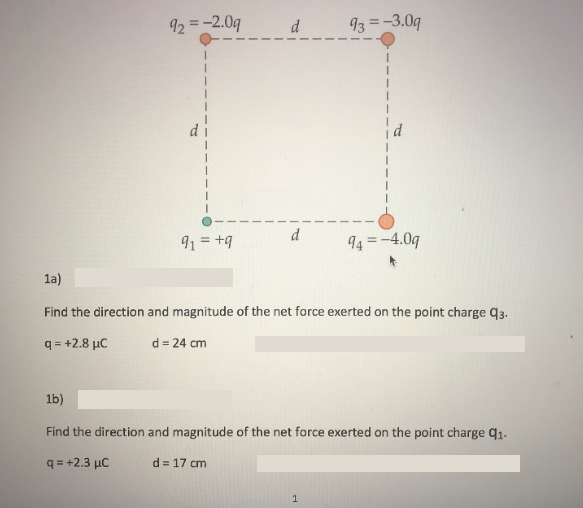# 13 -3.0q 4-4.0q 1a) Find the direction and magnitude of the net force exerted on the point charge q3. q=+2.8pC d=24 cm Find the direction and magnitude of the net force exerted on the point charge q1. qs+2.3 pC d=17cm

Questionhelp_outlineImage Transcriptionclose13 -3.0q 4-4.0q 1a) Find the direction and magnitude of the net force exerted on the point charge q3. q=+2.8pC d=24 cm Find the direction and magnitude of the net force exerted on the point charge q1. qs+2.3 pC d=17cm fullscreen

### Want to see the step-by-step answer?